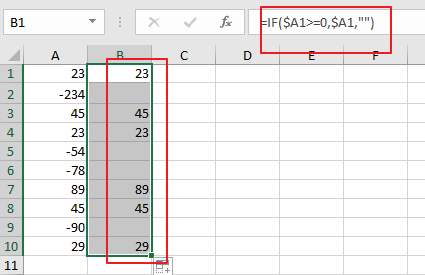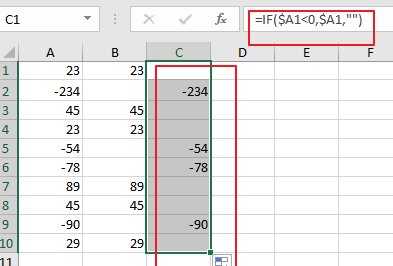# How To Split Columns to Seprated Negative and Positive Values in Excel

This post will guide you how to separate negative numbers and positive numbers from a column into two columns with a formula in Excel. How do I split numbers into two columns with negative and positive numbers in Excel.

## 1. Splitting Columns to Separated Positive and Negative Numbers

Assuming that you have a list of data in which contain positive and negative numbers in column A in your worksheet, and you want to separate your data into two columns. and you want to column B to list all positive nubmers from column A, and column C to show all negative numbers from column A. How to do it. and you need to use two formula based on the IF function. see below:

Extracting all Positive numbers:

``=IF(\$A1>=0,\$A1,"")``

You need to type this formula into Cell B1, and press `Enter` key on your keyboard, and then drag the AutoFill Handle down to other cells.Extracting All Negative Numbers:

``=IF(\$A1<0,\$A1,"")``You need to type this formula into Cell C1, and press `Enter` key on your keyboard, and then drag the AutoFill Handle down to other cells.

## 2. Video: Splitting Columns to Separated Positive and Negative Numbers

In this tutorial video, we will show you how to split a list of numbers into two columns based on positive and negative values in Excel.

## 3. Related Functions

• Excel IF function
The Excel IF function perform a logical test to return one value if the condition is TRUE and return another value if the condition is FALSE. The IF function is a build-in function in Microsoft Excel and it is categorized as a Logical Function.The syntax of the IF function is as below:= IF (condition, [true_value], [false_value])….